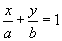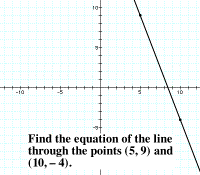index: click on a letter A B C D E F G H I J K L M N O P Q R S T U V W X Y Z A to Z index index: subject areas numbers & symbols sets, logic, proofs geometry algebra trigonometry advanced algebra & pre-calculus calculus advanced topics probability & statistics real world applications multimedia entrieswww.mathwords.com about mathwords website feedback

Equation of a Line

The various common forms for the equation of a line are listed below. In all forms, slope is represented by m, the x-intercept by a, and the y-intercept by b.

Note: The standard form coefficients A, B, and C have no particular graphical significance.

 Forms for the Equation of a Line Slope-intercept y = mx + b Used when you have the slope and the y-intercept. Point-slope y – y1 = m(x – x1) (x1, y1) is a point on the line. Standard form Ax + By = C If possible, A is nonnegative and A, B, and C are relatively prime integers. Two-interceptUsed when you have both intercepts. Vertical x = a All points have x-coordinate a. Horizontal y = b All points have y-coordinate b.

Movie Clips (with narration)Horizontal Line: how to find the equation (1.43M)Vertical Line: how to find the equation (1.46M)Point and Slope: How to find the equation of a line (4.13M)Two Points: How to find the equation of a line (5.5M)